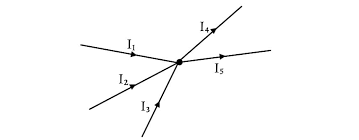# Kirchhoff's Current LawKirchhoff’s current law also known as Kirchhoff’s first law states that the algebraic sum of currents meeting at a junction of an electric circuits is zero. In the other hand it is simply means that the total current leaving a junction is equal to the total current entering that junction.

Symbolically

∑I=0

Explanation: let us consider 5 currents meeting t a junction P of the network as shown in figure.

Figure:Let us adopt the following sign conversions for determine the algebraic sign of different currents. All current entering the junction would be taken as positive whereas those leaving it would be taken as negative. According to the above convention, I1 and I4 would be taken as positive whereas I2, Iand I would be taken as negative. Using Kirchhoff’s of current law we have,

I1+(-I2)+(-I3)+I4 +(-I5)=0

Or, ∑I=0

Also, taking the negative of the expression on one side and positive sign on other side,

I1+ I4=I2+I3+I5

Incoming current =outgoing current

Iin=Iout.

Gustav Robert Kirchhoff was a German physicist who contributed to the fundamental understanding of electrical circuits, spectroscopy, and the emission of black-body radiation by   heated objects. So the pronunciation is quite different than what we usually hear..

and here is a video about the law.